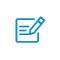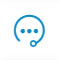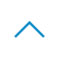# 用PyTorch实现一个简单的分类器1，自定义生成一个训练集，具体为在二维平面上的一些点，分为两类；

2，构建一个浅层神经网络，实现对特征的拟合，主要是明白在 pytorch 中网络结构如何搭建；

3，完成训练和测试部分的工作，熟悉 pytorch 如何对网络进行训练和测试。

1. 自定义生成数据集

1. n_data = torch.ones(100, 2)
2. x0 = torch.normal(2*n_data, 1)
3. y0 = torch.zeros(100)
4. x1 = torch.normal(-2*n_data, 1)
5. y1 = torch.ones(100)
6. x = torch.cat((x0, x1)).type(torch.FloatTensor)
7. y = torch.cat((y0, y1)).type(torch.LongTensor)

2. 构建一个浅层神经网络

1. class Net(torch.nn.Module):
2.     def __init__(self, n_feature, n_hidden, n_output):
3.         super(Net, self).__init__()
4.         self.n_hidden = torch.nn.Linear(n_feature, n_hidden)
5.         self.out = torch.nn.Linear(n_hidden, n_output)
6.     def forward(self, x_layer):
7.         x_layer = torch.relu(self.n_hidden(x_layer))
8.         x_layer = self.out(x_layer)
9.          x_layer = torch.nn.functional.softmax(x_layer)
10.         return x_layer
11. net = Net(n_feature=2, n_hidden=10, n_output=2)
12. # print(net)
13. optimizer = torch.optim.SGD(net.parameters(), lr=0.02)
14. loss_func = torch.nn.CrossEntropyLoss()

optimizer 就是这里定义的优化方式，其中的 lr 是学习率的参数。然后损失函数我们选择交叉熵损失函数，也就是上面的最后一行代码。优化算法和损失函数，可以在 pytorch 中直接选择不同的 api 接口，形式上直接参考上面这种固定形式便可。

3. 完成训练和测试

1. for i in range(100):
2.     out = net(x)
3.     # print(out.shape, y.shape)
4.     loss = loss_func(out, y)
6.     loss.backward()
7.     optimizer.step()

1. # train result
2. train_result = net(x)
3. # print(train_result.shape)
4. train_predict = torch.max(train_result, 1)
5. plt.scatter(x.data.numpy()[:, 0], x.data.numpy()[:, 1], c=train_predict.data.numpy(), s=100, lw=0, cmap='RdYlGn')
6. plt.show()1. # test
2. t_data = torch.zeros(100, 2)
3. test_data = torch.normal(t_data, 5)
4. test_result = net(test_data)
5. prediction = torch.max(test_result, 1)
6. plt.scatter(test_data[:, 0], test_data[:, 1], s=100, c=prediction.data.numpy(), lw=0, cmap='RdYlGn')
7. plt.show()扫码入群咨询反馈返回顶部